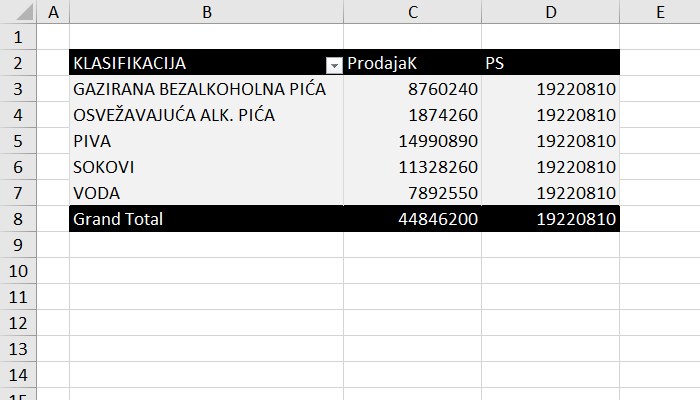# Belonging to a set

If we want to check, within the DAX statement, whether the element belongs to the given set we will use the operator IN and then, in the extension, in curly brackets, list the elements that belong to the set. In this way we can associate a filter within the CALCULATE function that specifies more than one value. A similar effect is achieved with the CONTAINSROW function.

As you know, when you want to specify the conjunction of two expressions in DAX, you will use the operator &&, and for the disjunction the operator ||. The NOT operator is used for negation, and the IN set is used to check the affiliation. If we check a condition that contains more than one value we can specify several conditions by joining them with the && operator. Also, we can use IN operator:

<expression> IN {<value 1>, <value 2>, … <value n>}

If we have created a statement that calculates the sum of all quantities sold using the formula:

ProdajaK:=SUM(Transakcije[KOL])

if we want to see the sum of quantities sold exclusively for the elements of the classification SOKOVI (FRUIT JUICES) and VODA (WATER) we will write the formula:

PS:=CALCULATE([ProdajaK],Artikli[KLASIFIKACIJA] IN {“VODA”,”SOKOVI”})We achieve exactly the same effect with the CONTAINSROW function. It has two arguments. The first is a set of values, and the second is a column that we filter. Let’s write the formula:

PS2:=CALCULATE([ProdajaK],CONTAINSROW({“VODA”,”SOKOVI”};Artikli[KLASIFIKACIJA]))